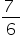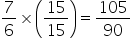Maths-
General
Easy

Question

# Identify 7:6 and 105:90 are equivalent ratios

## The correct answer is: Yes

### Equivalent ratios can be fin a by multiplying or dividing the parts of ratio by the same number. Hore7:6 =can be simplified further.Multiplying by same number7:6 and 105:90 are equivalent ratios#### With Turito Foundation.#### Get an Expert Advice From Turito.